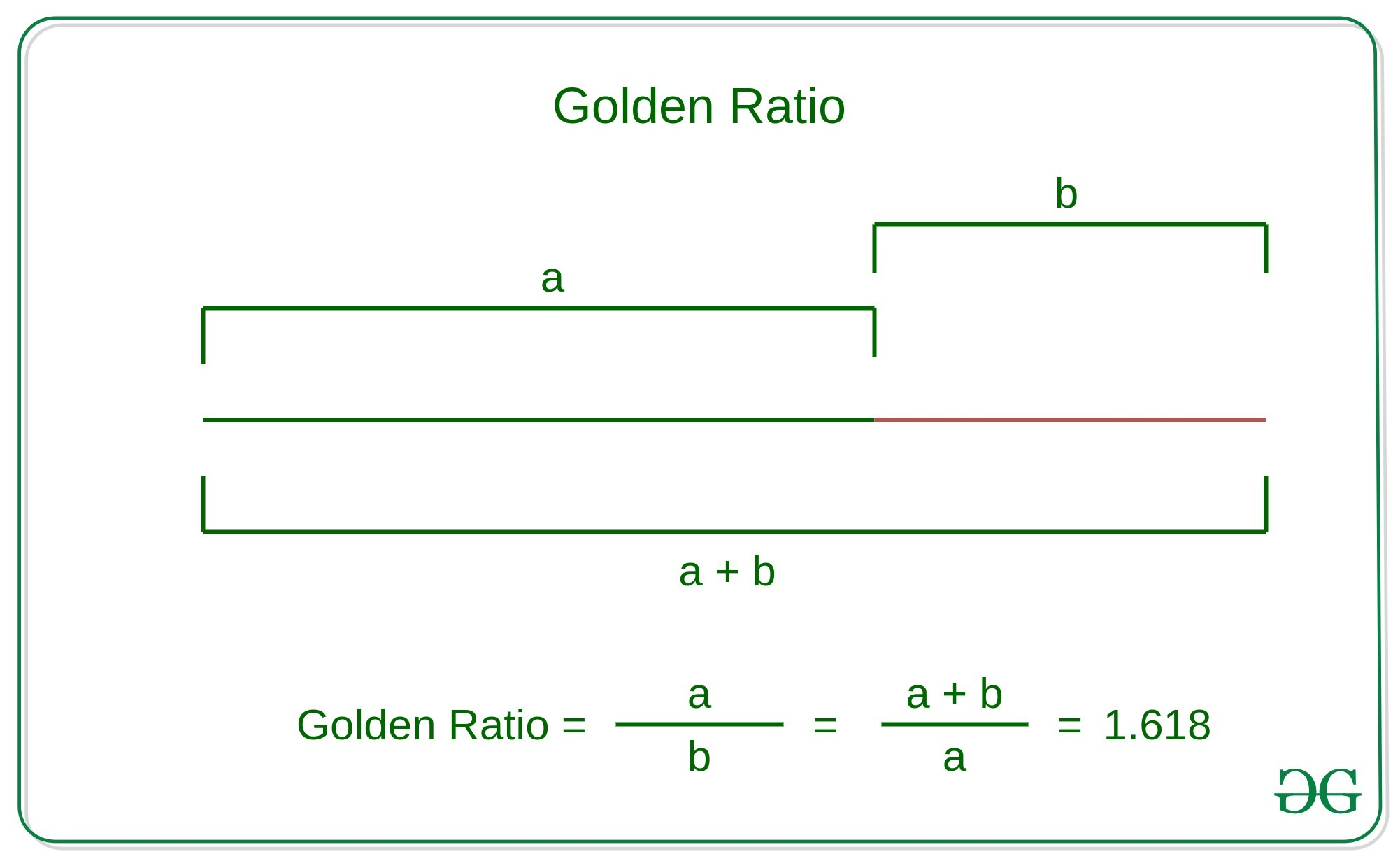# Check whether two numbers are in golden ratio

Given two numbers A and B, the task is to check that A and B are in golden ratio.

Golden Ratio: Two numbers are said to be in golden ratio if their ratio is same as the ratio of the sum of the two numbers to the larger number. Here a > b > 0, Below is the geometric representation of the Golden ratio:Examples:

Input: A = 1, B = 0.618
Output: Yes
Explanation:
These two numbers together forms Golden ratioInput: A = 61.77, B = 38.22
Output Yes
Explanation:
These two numbers together forms Golden ratio## Recommended: Please try your approach on {IDE} first, before moving on to the solution.

Approach: The idea is to find two ratios and check that these ratio is equal to the Golden ratio. That is 1.618.

// Here A denotes the larger numberBelow is the implementation of the above approach:

## Python

 # Python implementation to check   # whether two numbers are in   # golden ratio with each other     # Function to check that two   # numbers are in golden ratio  def checkGoldenRatio(a, b):             # Swapping the numbers such       # that A contains the maximum      # number between these numbers      a, b = max(a, b), min(a, b)             # First Ratio      ratio1 = round(a/b, 3)             # Second Ratio      ratio2 = round((a+b)/a, 3)      # Condition to check that two      # numbers are in golden ratio      if ratio1 == ratio2 and\         ratio1 == 1.618:          print("Yes")          return True     else:          print("No")          return False            # Driver Code  if __name__ == "__main__":      a = 0.618     b = 1            # Function Call      checkGoldenRatio(a, b)

Output:

Yes


References: https://en.wikipedia.org/wiki/Golden_ratio

Attention reader! Don’t stop learning now. Get hold of all the important DSA concepts with the DSA Self Paced Course at a student-friendly price and become industry ready.

My Personal Notes arrow_drop_upIf you like GeeksforGeeks and would like to contribute, you can also write an article using contribute.geeksforgeeks.org or mail your article to contribute@geeksforgeeks.org. See your article appearing on the GeeksforGeeks main page and help other Geeks.

Please Improve this article if you find anything incorrect by clicking on the "Improve Article" button below.

Article Tags :
Practice Tags :

2

Please write to us at contribute@geeksforgeeks.org to report any issue with the above content.